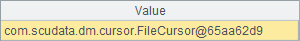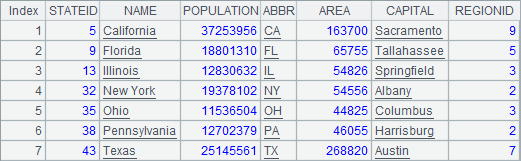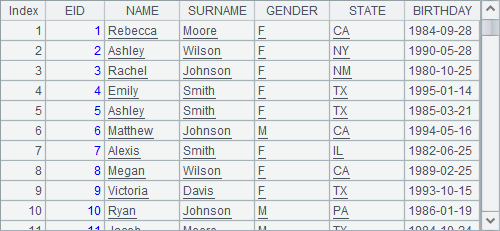# 内置并行

## 并行读取数据

 A B 1 =file("PersonnelInfo.txt") =now() 2 =A1.import@t() =now() 3 =A1.import@mt() =now() 4 =string(interval@ms(B1,B2))+"/"+string(interval@ms(B2,B3))

A2中直接读入数据，A3中添加了@m选项，使用f.import@m()，多线程读取数据。在A4中比较了两种方法的耗时，结果如下：## 有序归并游标

 A B 1 =file("Order_Wines.txt").cursor@t() =file("Order_Foods.txt").cursor@t() 2 =file("Order_Electronics.txt").cursor@t() =file("Order_Books.txt").cursor@t() 3 =[A1:B2].mergex(Date) =A3.fetch(10000) 4 >A3.close()

A3中，将4个文本中的订单数据根据日期有序归并，得到新的游标。

B3中取出前10000条数据如下：